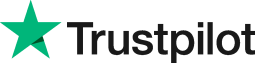Excel Round Function
• Home
• Courses
• Promotions
• Schedule
• Formats
• Our Clients

Forum home » Topics » Excel Round Function

# Excel Round Function

In business it is often helpful to round up or down a calculation to have fewer or more decimal places in your spreadsheet. This is done not just to make figures easier to read but also to ensure rounded calculations are displayed precisely.

For example, suppose a summary balance sheet results in the following figures:

Total Income £25,354.54
Total Expenses £5,643.11

Suppose you wish to calculate the Net Profit (Total Income - Total Expenses) but all figures are to be rounded to the nearest pound.

If you simply format the figures in Excel using Format Cells, Number and set the decimal places to zero you will see the following:

Total Income £25,355
Total Expenses £35,643
Net Profit £19,711

But someone might ask where the missing pound has gone!

The reason is the figures are not truly rounded but only appear that way. The net profit is still doing the calculation on the values with 2 decimal places.

The way to truly round the numbers is to use the ROUND function. For example if the Total Income is in B1 then in C1 type =Round(B1,0)

Copying down the formula results in the correctly rounded figures with no missing pounds.

£25,355
£5,643
£19,712

The ROUND function allows you to round a number to a specified number of digits. For example, if cell A1 contains 123.7825, and you want to round that value to two decimal places, you can use the following formula:

=ROUND(A1, 2)
The result of this function is 123.78.

=ROUND(A1,1)
This return a value of 123.8 - as the number 8 is more than 5.

=ROUND(A1,0)
This will round up and displays 124 in the cell.

=ROUND(A1,-1)
This will round down and display 120 in the cell because 3, in the ones position, is less than 5.

=ROUND(A3,-2)
This will round up and display 100 in the cell since 2, in the tens position, is less than 5.

See below for further information related to rounding numbers with Excel function.

# Related forum posts:

## Rounding numbers

Hi, Can you tell me if it is possible to round numbers on excel to the next 50p? I realise that this is not the conventional way to round numbers. If, for example, a number is 34.02 I need it to round up to 34.50. Also, if a number appears as 56.73 I need it to round up to 57.00. Is this possible and, if so, how do I do it? Many thanks! Emma

## Rounding numbers

How do I round off large numbers in excel ?

# Related articles

## Some Simple Yet Less Common Excel Functions

I love that Excel can do so many things so quickly and since there are hundreds of functions I am always coming across ones I was completely unaware of. In this article I have compiled a few of my favourite, less commonly used functions.

 Welcome. Please choose your application (eg. Excel) and then post your question. Our Microsoft Qualified trainers will then respond within 24 hours (working days). Frequently Asked Questions What does 'Resolved' mean? Any suggestions, questions or comments? Please post in the Improve the forum thread.TrustScore 4.9 / 5       1244 reviews